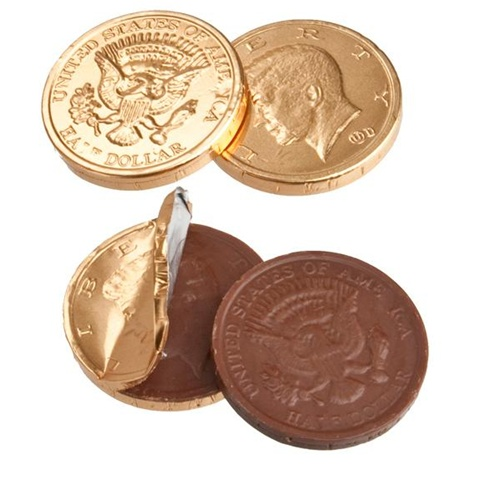# The Four Chocolate Coins

Probability Level 4Alexane has four chocolate fair coins.
She flips all the coins and eats those that fall on head.
She flips the coins not eaten (those that fell on tail) a second time and eats those that fall on head.

What is the probability that Alexane has eaten more than 2 coins?

If the probability can be expressed as $\dfrac ab$, where $a$ and $b$ are coprime positive integers, find $a+b$.

×